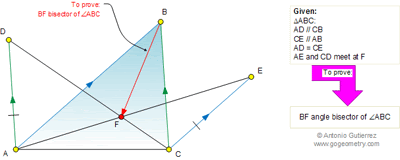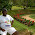## Saturday, June 18, 2011

### Problem 625: Triangle, Parallel Lines, Congruence, Angle Bisector

Geometry Problem
Level: Mathematics Education, High School, Honors Geometry, College.

Click the figure below to see the complete problem 625.1.http://img31.imageshack.us/img31/8388/problem625.png
Let CD cut AB at M and AE cut BC at L and angle bisector of angle ABC cut AC at N ( see picture)
we have LB/LC= x/c ( tri. LAB similar to Tri. LEC)
MB/MA=a/x ( Tri. MAD similar to tri. MBC)
NA/NC= c/a ( BN is angle bisector of angle(ABC))
Note that LB/LC . NA/NC . MC/MA= x/c .c/a .a/x= 1
So CM, AL and BN concurrent at F per Ceva's theorem and BF is angle bisector of angle ABC
Peter Tran

2.Denote intersection of
AF, BC by P;
BF, AC by Q;
CF, AB by R.
(AQ/QC)(CP/PB)(BR/RA) = 1 by Ceva.
Consider triangle AFC and point B.
BF intersects AC at Q; BA intersects CF at R; BC intersects AF at P
Again by Ceva, (AQ/QC)(CR/RF)(FP/PA) = 1
It follows from the above two equations that
(CP/PB)(BR/RA)= (CR/RF)(FP/PA)
Now AB // CE implies triangles CPE and BPA are similar and so
CP/PB = CE/AB
Similarly CB//AD implies triangles BRC and ARD are similar and so
Multiplying the last two equations,
Thus (CR/RF)(FP/PA) = BC/AB
Now denote the areas BFC, CFA, AFB by x, y, z respectively
Clearly
CR/RF = (BRC) /(BRF)
= (ARC)/(ARF)
= [(BRC) + (ARC)] /[(BRF) + (ARF)]
=(ABC)/(AFB)
Similarly,
FP/PA = (BFC)/(ABC)
Thus (CR/RF)(FP/PA)= (BFC) /(AFB) = CQ/QA
Follows CQ/QA = CB/BA
Hence BF(Q) bisects angle ABC

3.Call intersection of DC and AB to be X, and intersection of AE and BC to be Y. AX/XB=DA/BC, and BY/YC=AB/CE. By Ceva, if BF cuts AC at point Z, then CZ/ZA*AX/XB*BY/YC=CZ/ZA*DA/BC*AB/CE=CZ/ZA*AB/BC=1, so CZ/ZA=BC/AB. By angle bisector theorem, BF must bisect <ABC.

4.Let AE, BC cut at X.

Let AD = CE = p, AF = m, FX = n, BX = t, CX = s.

From similar triangles,

p/s = m/n …(1) and
p/c = s/t ….(2)

From (1) and (2), m/n =c/t and so BF bisects <B

Sumith Peiris
Moratuwa
Sri Lanka

5.In the quadrilateral ABCD, AB=AD, <ABC=<ADC =90⁰. On the sides BC and CD are assigned respectively the points M and N on each sides of the triangle, such that DM⊥AN. Find that AM⊥BN

Erina NJ

1.Hi Erina,